Reconstruction of the 2014 Harewell Lane formation 1.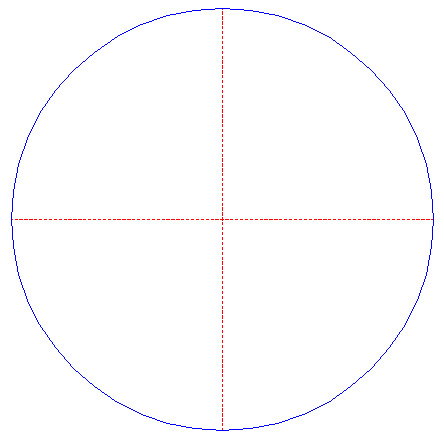Draw a circle. Draw the horizontal and vertical centerlines. 2.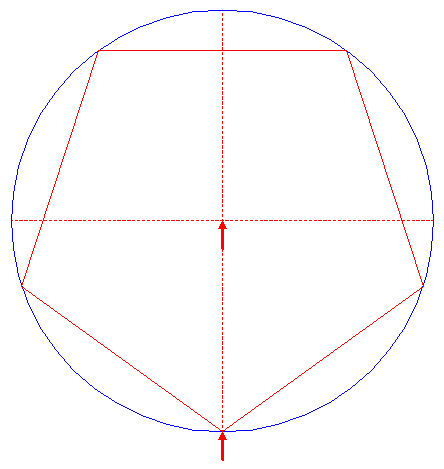Construct the inscribed pentagon (regular 5-sided polygon) of cirle 1, pointing down. 3.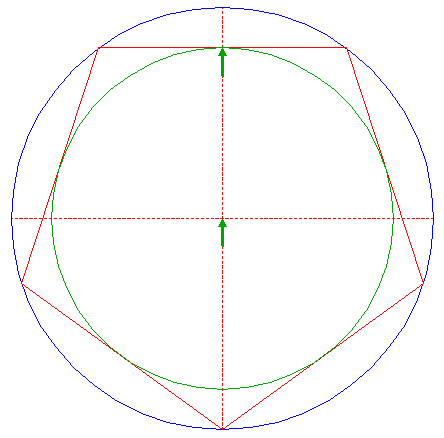Construct the inscribed circle of pentagon 2. 4.Construct the inscribed pentagon of cirle 3, pointing up. 5.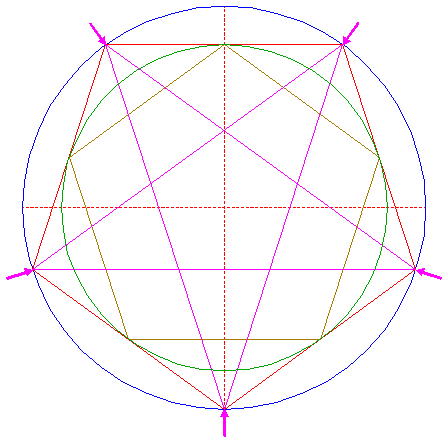Construct the inscribed pentagram (regular 5-pointed star) of pentagon 2, by drawing all diagonals. 6.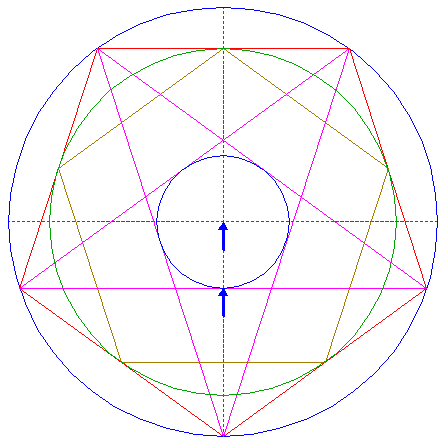Construct the inscribed circle of the pentagon enclosed by pentagram 5. 7.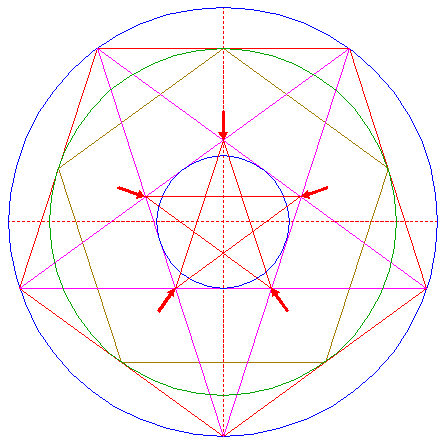Construct the inscribed pentagram of the pentagon enclosed by pentagram 5. 8.Construct the inscribed circle of the pentagon enclosed by pentagram 7. 9.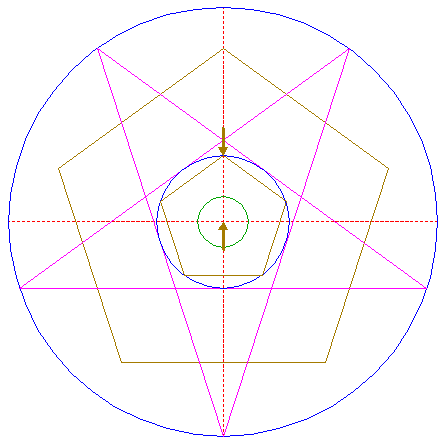Construct the inscribed pentagon of cirle 6, pointing up. 10.Construct a circle concentric to circle 1, passing through the righthand intersection of pentagon 9 and the horizontal centerline. 11.Construct a circle, tangent to the lower lefthand and righthand sides of pentagram 5, and tangent to the lower side of pentagon 4 from below, as shown. 12.Copy circle 11 to the lower intersection of circle 1 and the vertical centerline. 13.Construct a circle concentric to circle 1, tangent to circle 12 at the upper side. 14.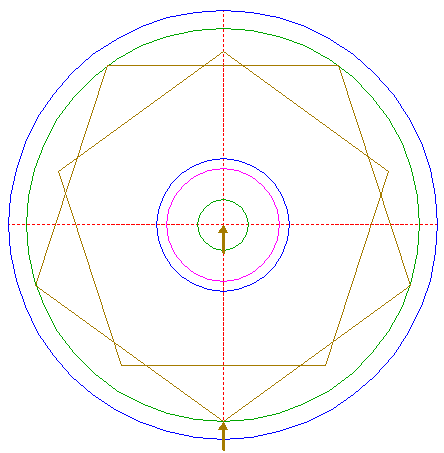Construct the inscribed pentagon of circle 13, pointing down. 15.Construct the inscribed pentagram of pentagon 14. 16.Construct a circle centered at the upper intersection of circle 10 and the vertical centerline, tangent to circle 6 at the upper side. 17.Copy circle 16 to the intersection of the horizontal side of pentagram 15 and the vertical centerline. 18.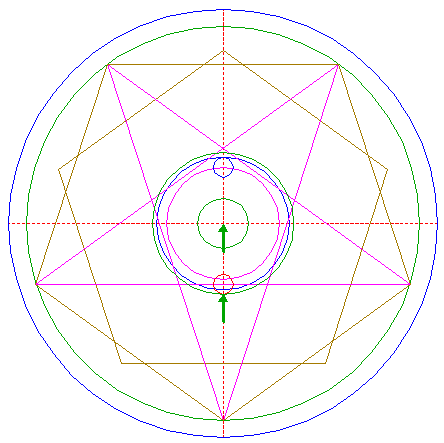Construct a circle concentric to circle 1, tangent to circle 17 at the lower side. 19.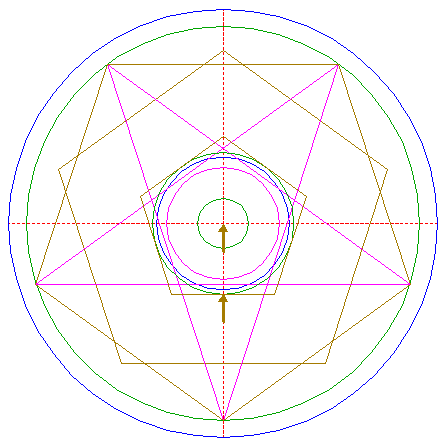Construct the circumscribed pentagon of circle 18, pointing up. 20.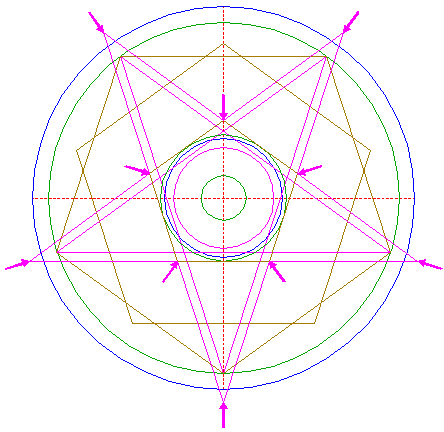Extend all sides of pentagon 19 in both directions, until they meet, just outside circle 1, as shown. 21.Circles 1, 6, 8, and 10, pentagon 4, pentagram 15, and lines 20, are used for the final reconstruction. 22.Remove all parts not visible within the formation itself. 23.Colour all areas corresponding to standing... 24....or to flattened crop, and finish the reconstruction of the 2014 Harewell Lane formation. 25.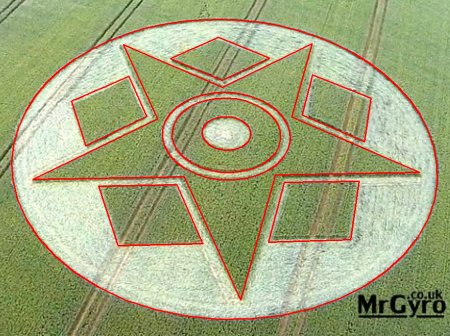The final result, matched with the aerial image.
 Copyright © 2014, Zef Damen, The Netherlands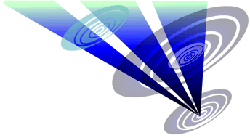BACKMark Fussell & Stuart Dike